Ex 5.1

Chapter 5 Class 10 Arithmetic Progressions (Term 2)
Serial order wise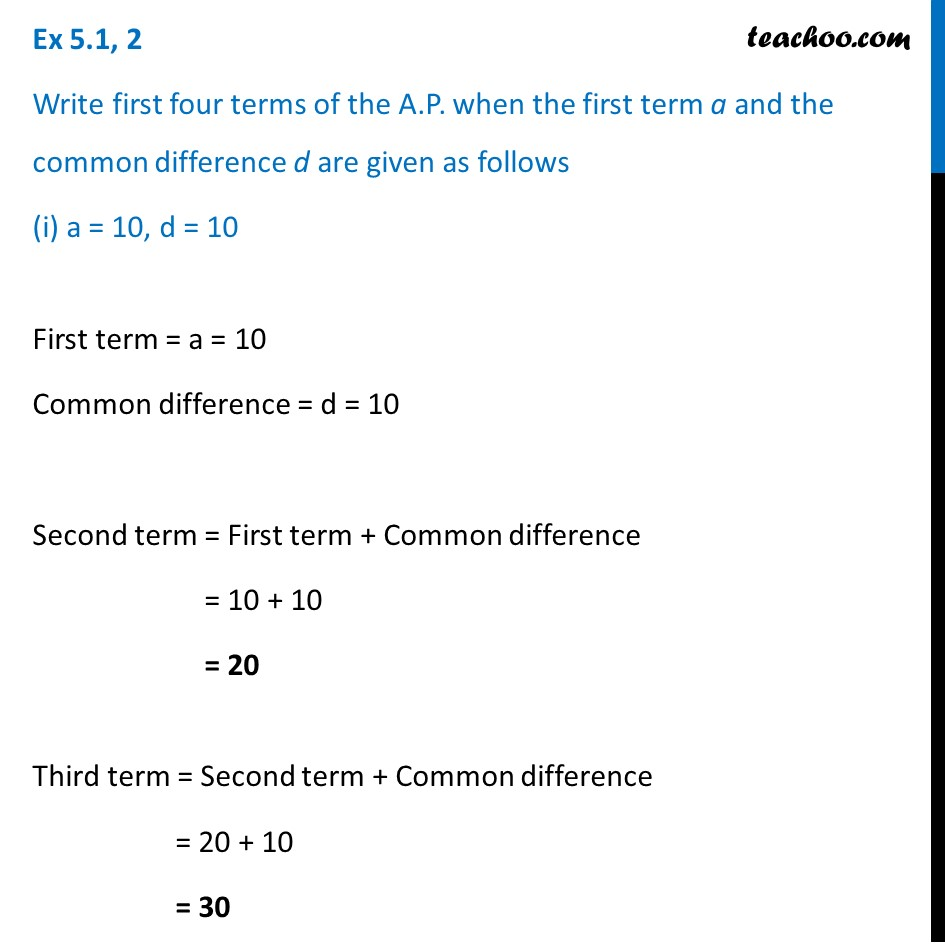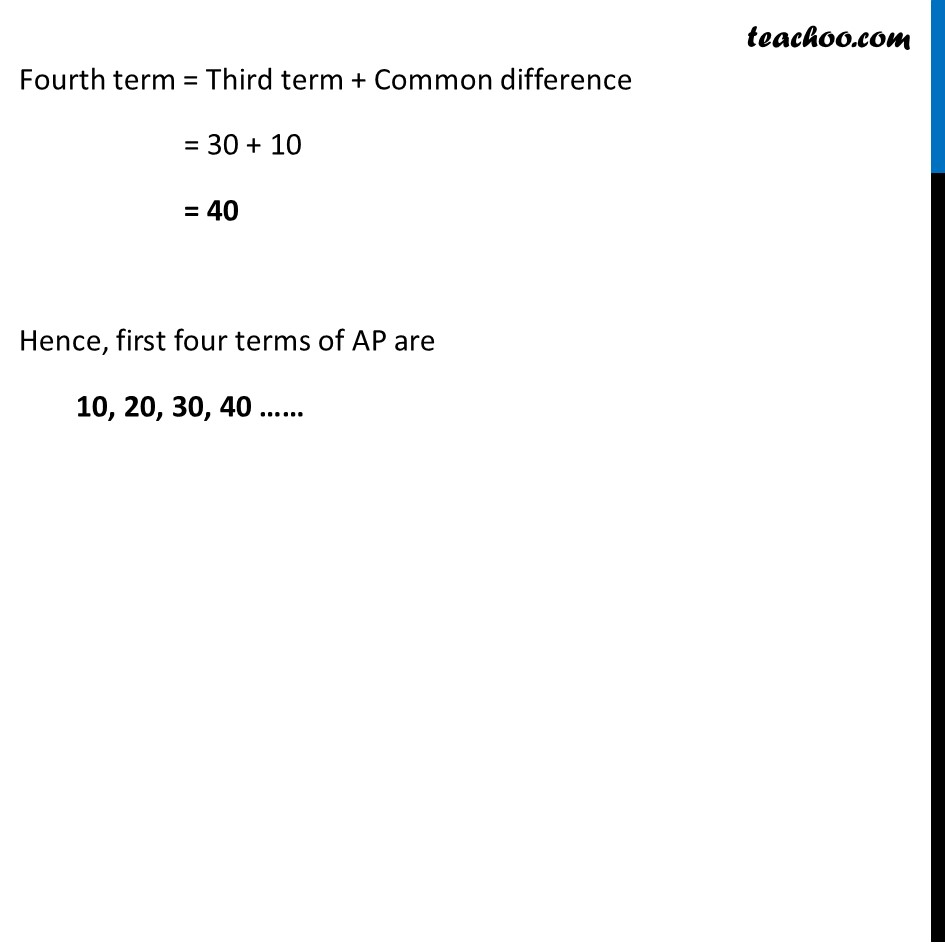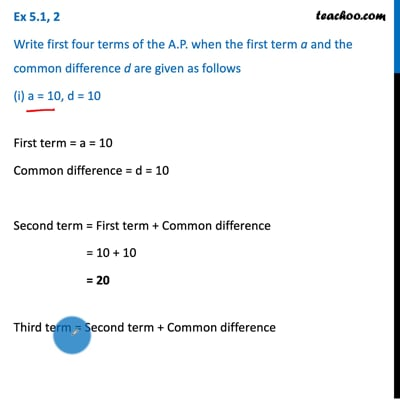This video is only available for Teachoo black users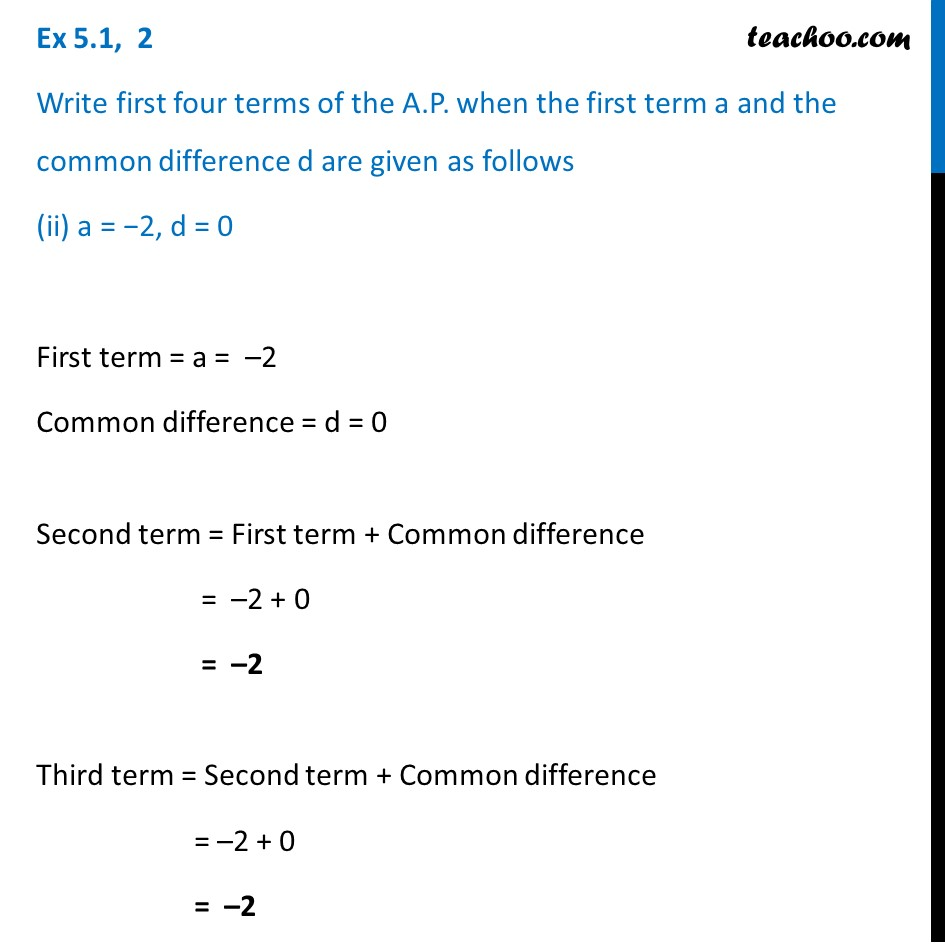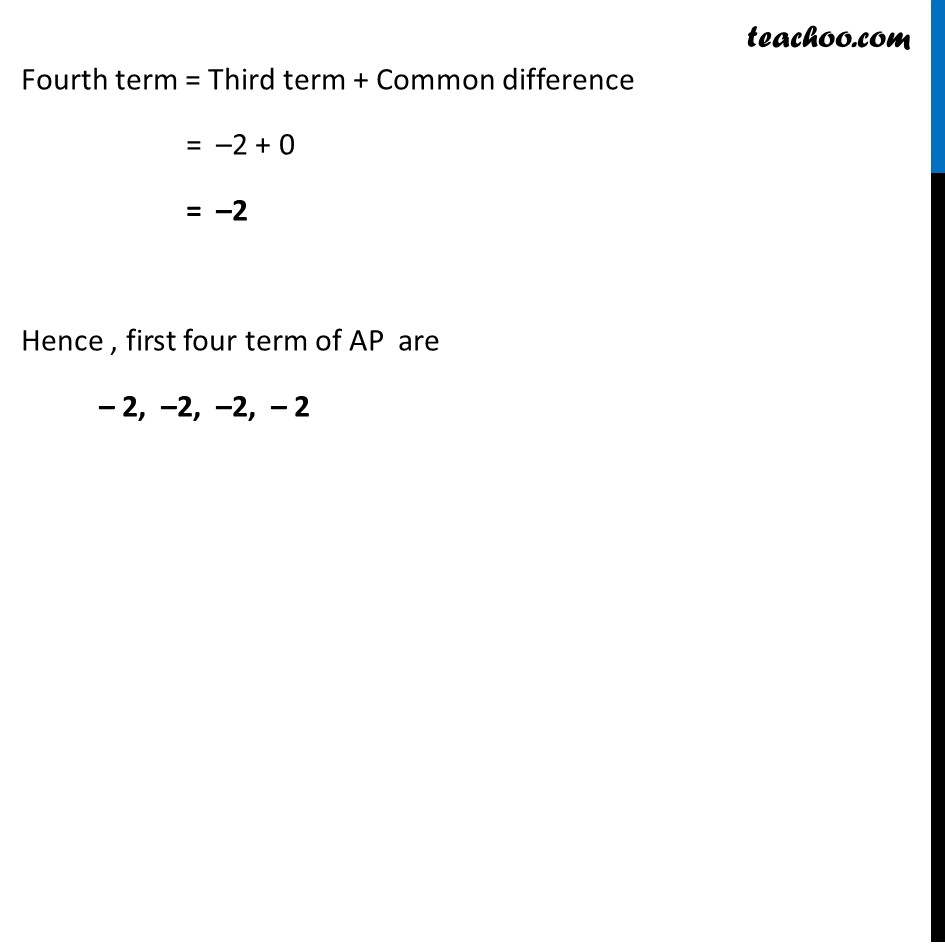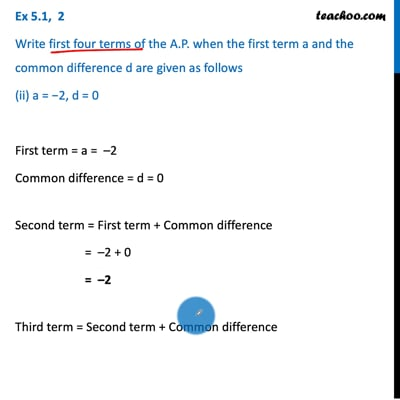This video is only available for Teachoo black users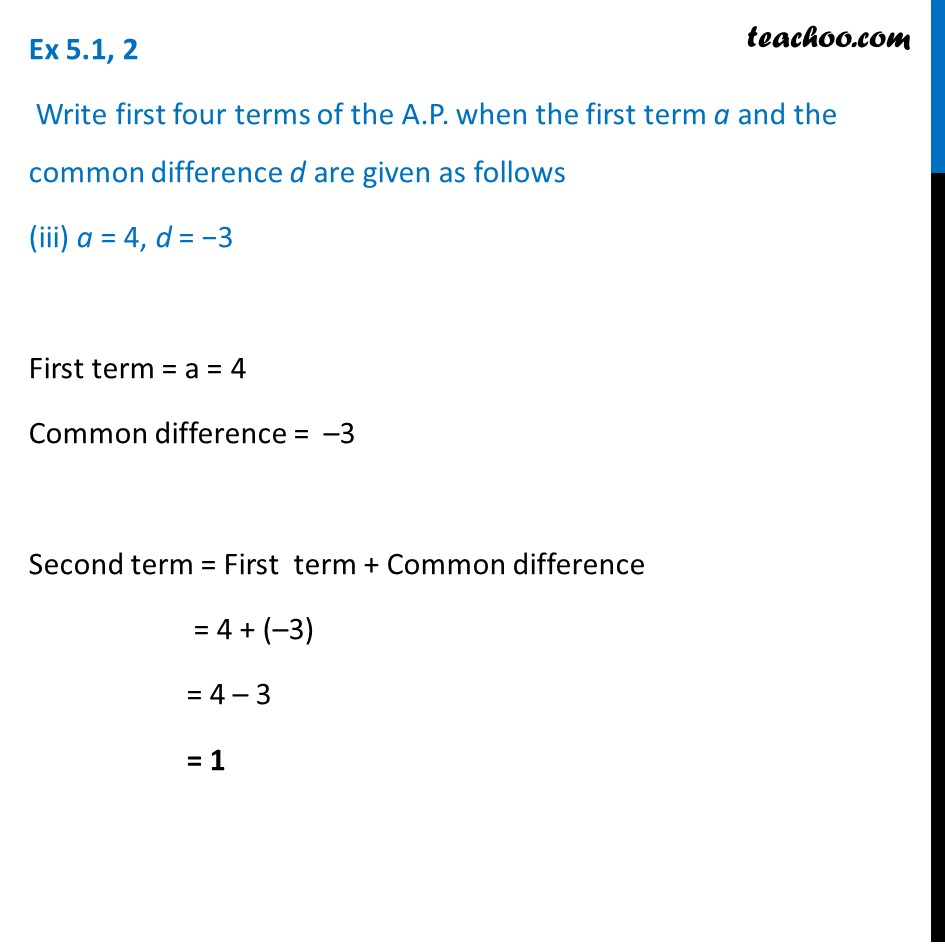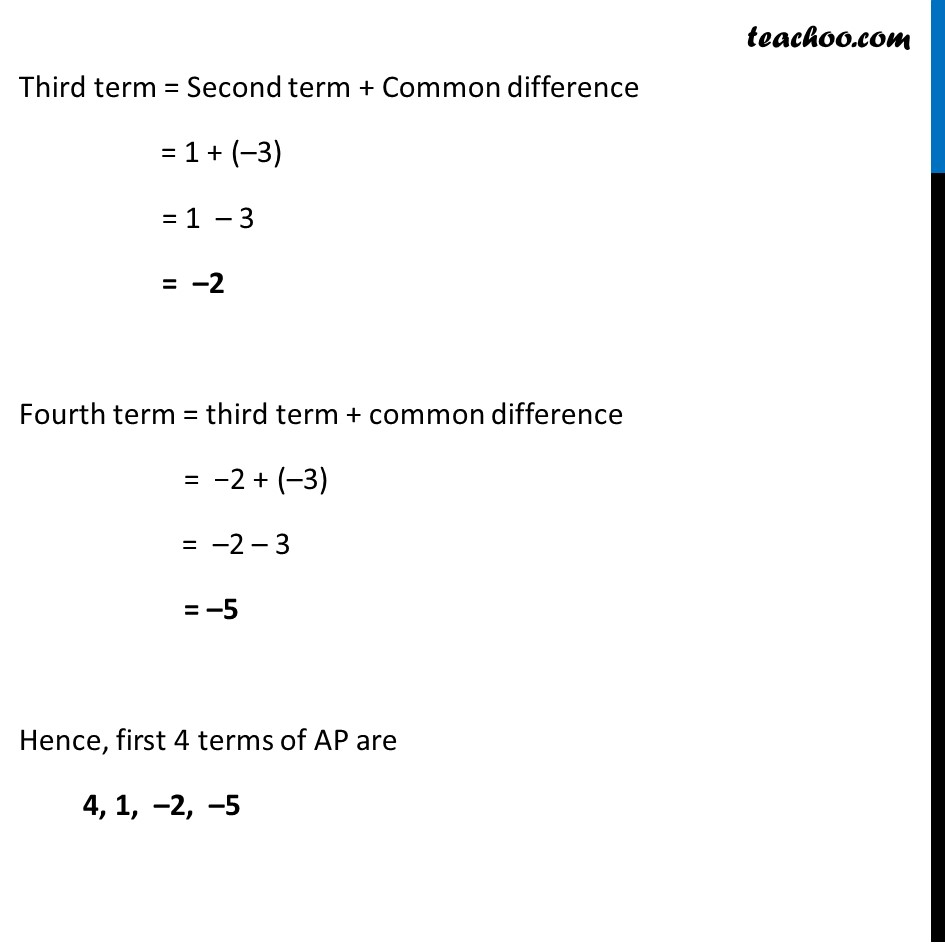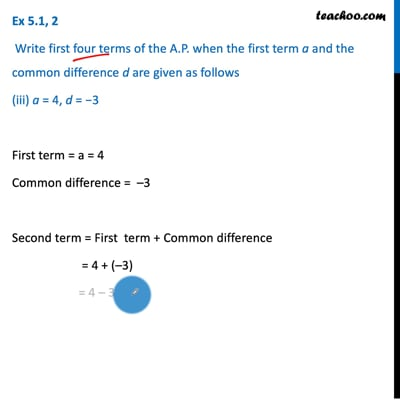This video is only available for Teachoo black users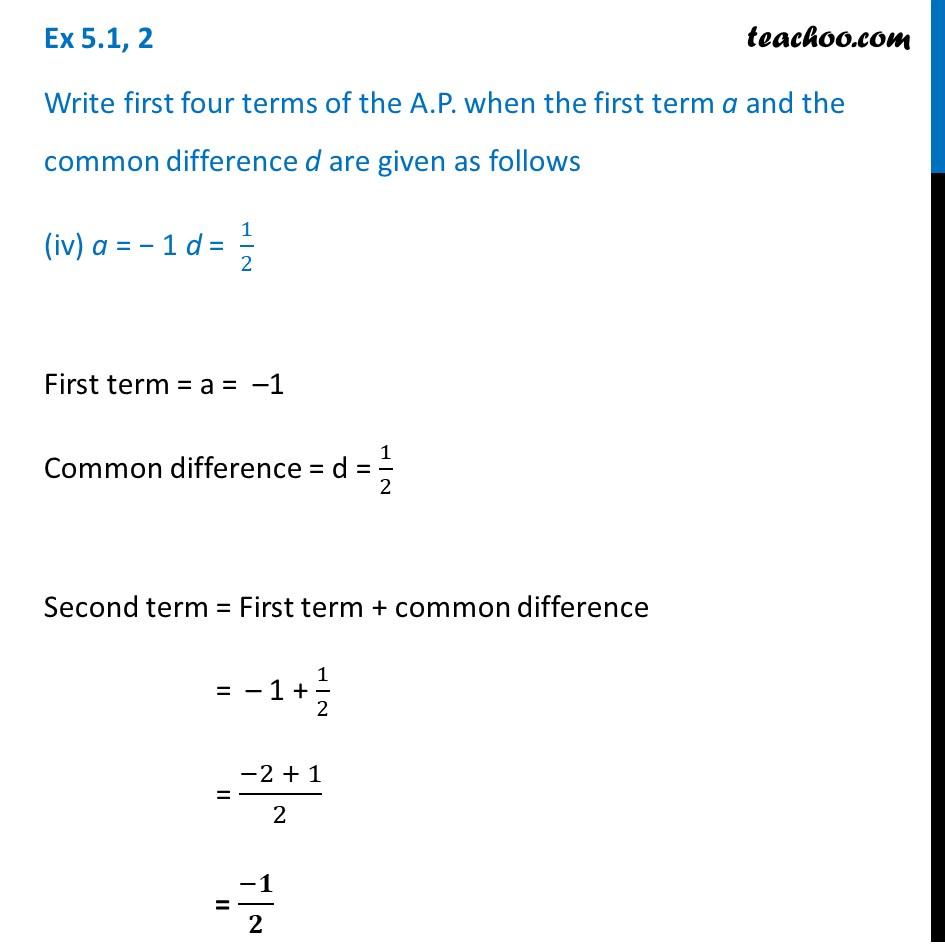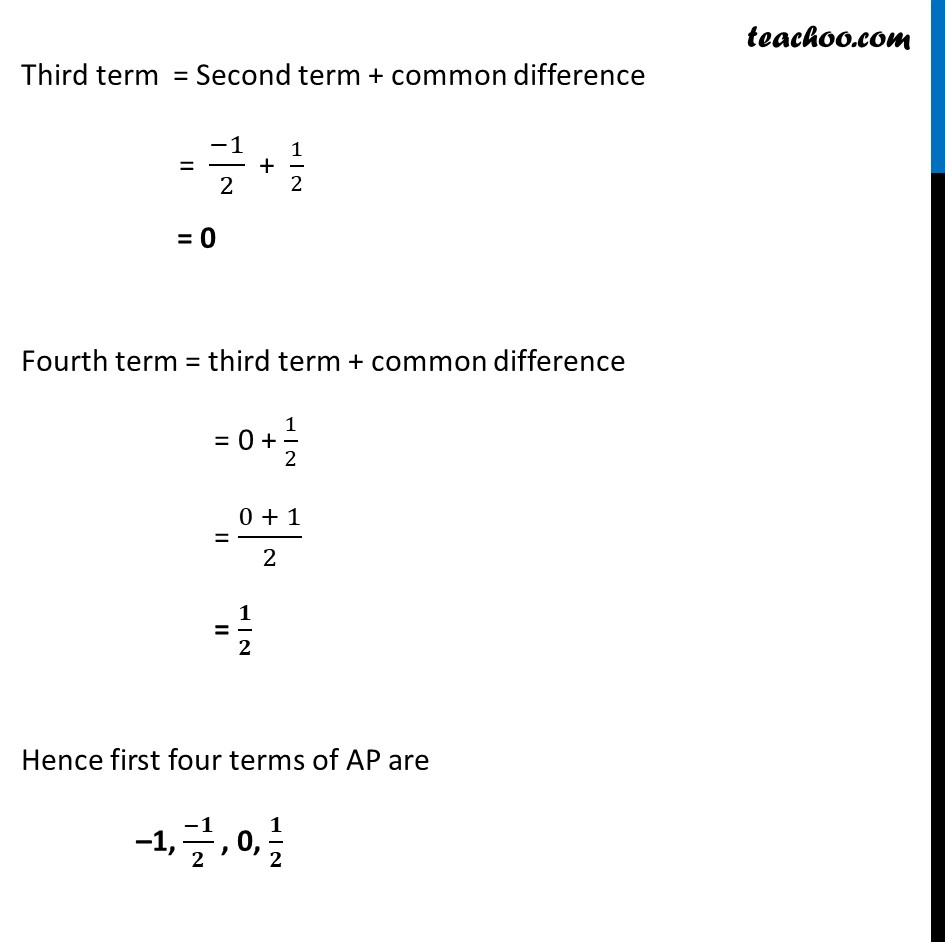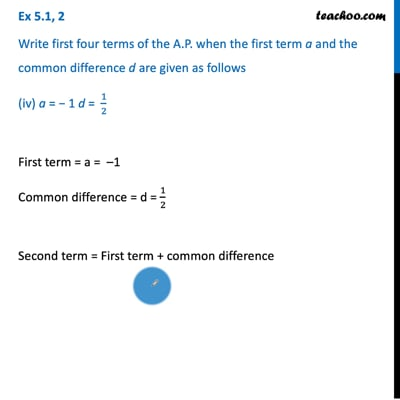This video is only available for Teachoo black users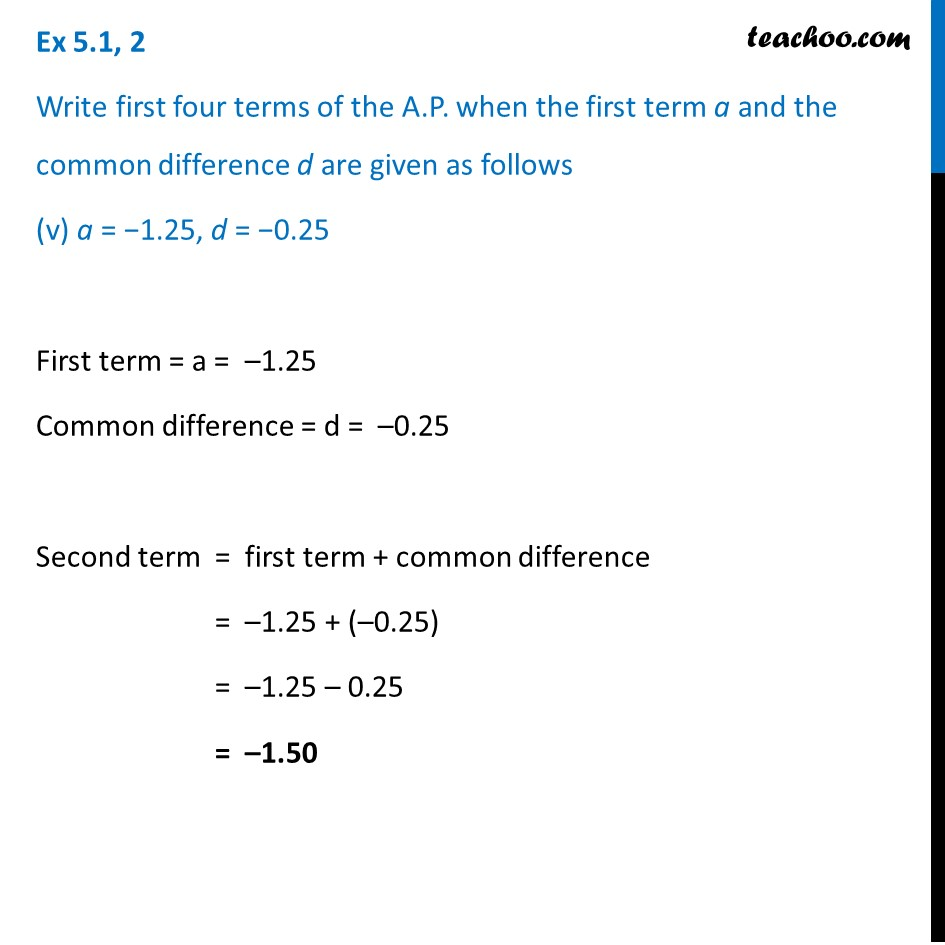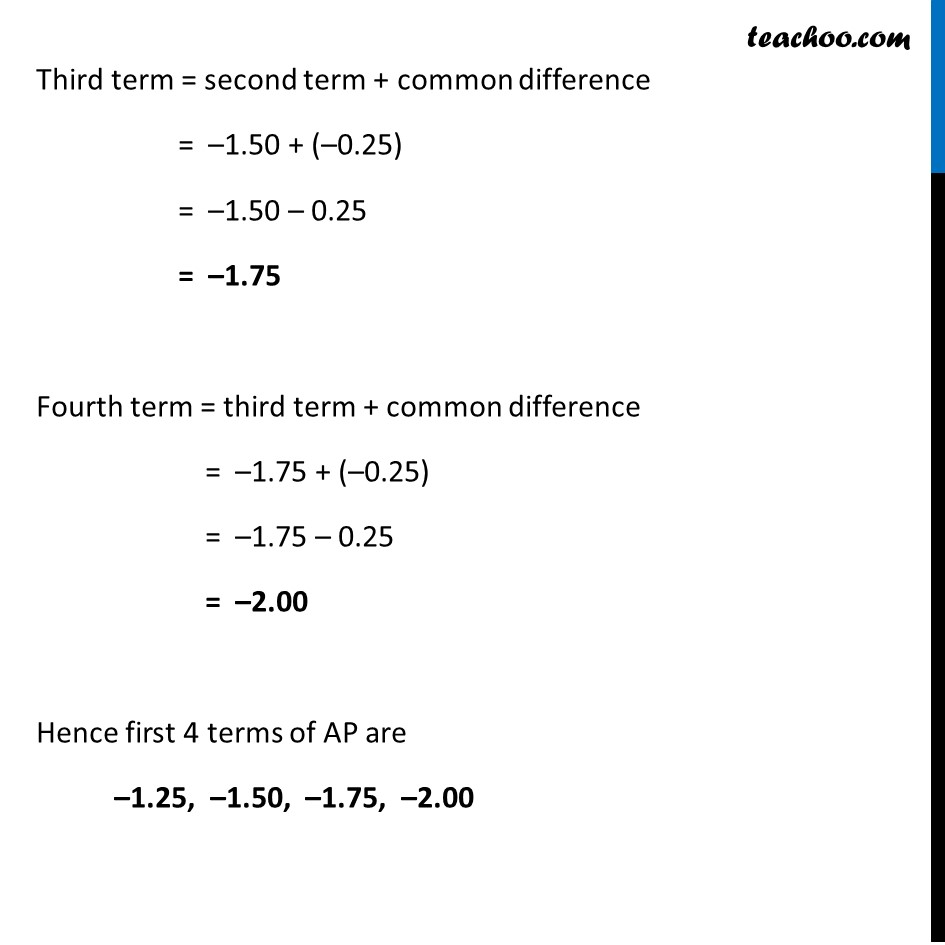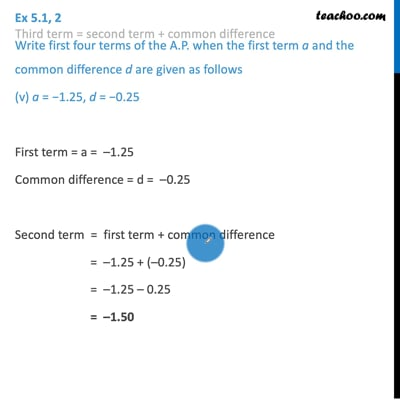This video is only available for Teachoo black users

### Transcript

Ex 5.1, 2 Write first four terms of the A.P. when the first term a and the common difference d are given as follows (i) a = 10, d = 10 First term = a = 10 Common difference = d = 10 Second term = First term + Common difference = 10 + 10 = 20 Third term = Second term + Common difference = 20 + 10 = 30 Fourth term = Third term + Common difference = 30 + 10 = 40 Hence, first four terms of AP are 10, 20, 30, 40 …… Ex 5.1, 2 Write first four terms of the A.P. when the first term a and the common difference d are given as follows (ii) a = −2, d = 0 First term = a = –2 Common difference = d = 0 Second term = First term + Common difference = –2 + 0 = –2 Third term = Second term + Common difference = –2 + 0 = –2 Fourth term = Third term + Common difference = –2 + 0 = –2 Hence , first four term of AP are – 2, –2, –2, – 2 Ex 5.1, 2 Write first four terms of the A.P. when the first term a and the common difference d are given as follows (iii) a = 4, d = −3 First term = a = 4 Common difference = –3 Second term = First term + Common difference = 4 + (–3) = 4 – 3 = 1 Third term = Second term + Common difference = 1 + (–3) = 1 – 3 = –2 Fourth term = third term + common difference = −2 + (–3) = –2 – 3 = –5 Hence, first 4 terms of AP are 4, 1, –2, –5 Ex 5.1, 2 Write first four terms of the A.P. when the first term a and the common difference d are given as follows (iv) a = − 1 d = 1/2 First term = a = –1 Common difference = d = 1/2 Second term = First term + common difference = – 1 + 1/2 = (−2 + 1)/2 = (−𝟏)/𝟐 Third term = Second term + common difference = (−1)/2 + 1/2 = 0 Fourth term = third term + common difference = 0 + 1/2 = (0 + 1)/2 = 𝟏/𝟐 Hence first four terms of AP are –1, (−𝟏)/𝟐 , 0, 𝟏/𝟐 Ex 5.1, 2 Write first four terms of the A.P. when the first term a and the common difference d are given as follows (v) a = −1.25, d = −0.25 First term = a = –1.25 Common difference = d = –0.25 Second term = first term + common difference = –1.25 + (–0.25) = –1.25 – 0.25 = –1.50 Third term = second term + common difference = –1.50 + (–0.25) = –1.50 – 0.25 = –1.75 Fourth term = third term + common difference = –1.75 + (–0.25) = –1.75 – 0.25 = –2.00 Hence first 4 terms of AP are –1.25, –1.50, –1.75, –2.00